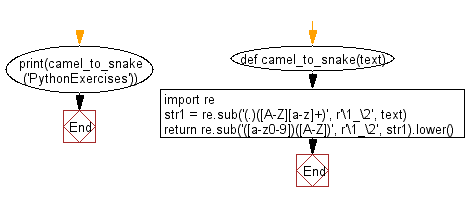﻿ Python: Convert camel case string to snake case string - w3resource# Python: Convert camel case string to snake case string

## Python Regular Expression: Exercise-36 with Solution

Write a python program to convert camel case string to snake case string.

Sample Solution:-

Python Code:

``````def camel_to_snake(text):
import re
str1 = re.sub('(.)([A-Z][a-z]+)', r'\1_\2', text)
return re.sub('([a-z0-9])([A-Z])', r'\1_\2', str1).lower()

print(camel_to_snake('PythonExercises'))
```
```

Sample Output:

```python_exercises
```

Pictorial Presentation:Flowchart:## Visualize Python code execution:

The following tool visualize what the computer is doing step-by-step as it executes the said program:

Python Code Editor:

Have another way to solve this solution? Contribute your code (and comments) through Disqus.

What is the difficulty level of this exercise?

Test your Python skills with w3resource's quiz

﻿

## Python: Tips of the Day

Getting the last element of a list:

some_list[-1] is the shortest and most Pythonic.

In fact, you can do much more with this syntax. The some_list[-n] syntax gets the nth-to-last element. So some_list[-1] gets the last element, some_list[-2] gets the second to last, etc, all the way down to some_list[-len(some_list)], which gives you the first element.

You can also set list elements in this way. For instance:

```>>> some_list = [1, 2, 3]
>>> some_list[-1] = 5 # Set the last element
>>> some_list[-2] = 3 # Set the second to last element
>>> some_list
[1, 3, 5]
```

Note that getting a list item by index will raise an IndexError if the expected item doesn't exist. This means that some_list[-1] will raise an exception if some_list is empty, because an empty list can't have a last element.

Ref: https://bit.ly/3d8TfFP